MCAT Physical : Submersion

Example Questions

← Previous 1 3 4

Example Question #1 : Fluids And Gases

Which of the following is true regarding Archimedes’ principle?

I. It states that the weight of an object is equal to the weight of liquid it displaces

II. It states that the buoyant force increases as the density of the object in the fluid increases

III. It is not applicable for an object that is completely submerged in a liquid

III only

I only

I and II

II and III

I only

Explanation:

Archimedes’ principle states that the upward buoyant force experienced by a solid object in a fluid is equal to the weight of the fluid displaced. In mathematical form, Archimedes’ principles states: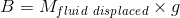Here,is the buoyant force,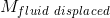is the mass of the fluid displaced, and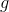is the acceleration due to gravity. Recall that density is defined as mass divided by volume; therefore, mass of fluid can be written as: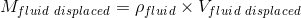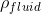is the density of fluid and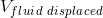is the volume of fluid displaced. Plugging this equation into Archimedes’ principle gives: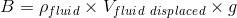Notice that the density of the object is not part of Archimedes’ principle; therefore, the the density of the object doesn’t affect the buoyant force.

Both submerged and floating objects in a fluid will experience an upward buoyant force that is equal to the weight of the fluid displaced; Archimedes’ principle is valid for both submerged and floating objects.

Example Question #2 : Fluids And Gases

A researcher has an object with a mass of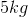and density of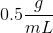. How many liters of water are displaced when the researcher places this object in water?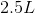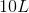Cannot be determined from the given information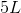Cannot be determined from the given information

Explanation:

To solve this question, you need to first compare the density of the object with the density of the fluid (water). The density of the object (according to the question) isand the density of water is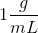. Since the density of the object is less than the density of water, the object will float. To solve for the volume of fluid displaced, we need to use Archimedes’ principle:Here,is buoyant force,is density of fluid,is volume of fluid displaced, andis acceleration due to gravity. We have two unknowns in this equation: the buoyant force and the volume of fluid displaced; therefore, we do not have enough information to solve for the volume of fluid displaced.

If the density of the object and the density of the fluid were equal, then we could have assumed that the weight of the fluid displaced (Buoyant force) equals the weight of the object. We could have then calculated the buoyant force and, subsequently, the volume of fluid displaced; however, we cannot make that assumption in this question because the density of the object is different from the density of the fluid.

Example Question #3 : Fluids And Gases

A student puts an object into a bowl of water. He observes that the object sinks to the bottom of the bowl. Based on this information, the student can conclude that the density of the object is __________ the density of water and the buoyant force on the object is __________ the weight of the water displaced.

less than . . . greater than

greater than . . . equal to

greater than. . . greater than

less than . . . equal to

greater than . . . equal to

Explanation:

The question states that the object sinks to the bottom of the bowl. Recall that objects with greater density than water will either submerge completely or sink, whereas objects with lesser density than water will float on the surface of water. The object in this question must have a greater density than water.

The second part of the question is asking you to compare the buoyant force with the weight of the fluid displaced. Archimedes’ principle states that the upward buoyant force always equals the weight of fluid displaced. Archimedes’ principle is valid for floating, submerging, and sinking objects; therefore, the buoyant force on the object will equal the weight of the water displaced.

Example Question #4 : Fluids And Gases

An object with a volume of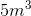has a density of. What volume of the object will be submerged if it is placed in water?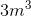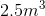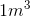Explanation:

The density of the object is, whereas the density of water is; therefore, the object will float at the surface of water. One of the implications of Archimedes’ principle for a floating object is that the percent of object submerged equals the ratio of the density of object to the density of fluid: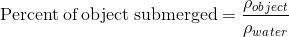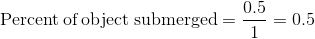This means that 50% of the object is submerged and 50% of the object is above the water. 50% of the volume of the object, or, is submerged.

Example Question #1 : Fluids And Gases

What is the density of a log that is 25cm long, has a cross sectional area of  5cm2, and weighs 100g?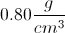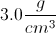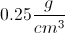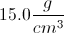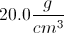Explanation:

The density of an object is equal to mass over volume.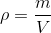Knowing the length and the cross sectional area of the log, we can find its volume.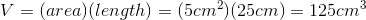Plugging in volume and mass into the equation will enable us to find density.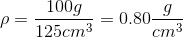Example Question #6 : Fluids And Gases

Solid A has a volume of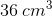and a density of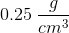. Solid B is cube with sides of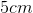and has a density of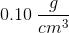.

What is the difference in mass between the two solids?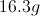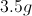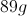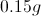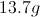Explanation:

The formula for density is: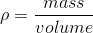In the question, we are given the densities of both solids and a means to find their volumes. Using these values, we will be able to determine the mass of each solid.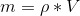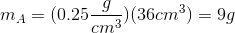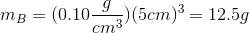Now that we know both masses, we can find the difference.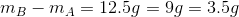Example Question #7 : Fluids And Gases

A researcher performs an elemental analysis on a compound. He finds that the compound is made up of only carbon, hydrogen, and oxygen atoms. He isolates a pure sample of the compound and finds that this sample contains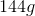of carbon,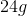of hydrogen, and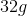of oxygen. The researcher wants to perform further analysis on this compound the next day. Before leaving the lab the researcher creates three stock solutions of varying concentrations of this compound: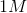(solution A),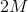(solution B), and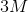(solution C). He stores these solutions overnight at a temperature of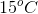.

Molecular weight of this compound =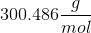Astock solution, solution X, kept at room temperature (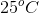) will have __________ compared to solution A.

the same density

a lower density

a greater density

a lower density

Explanation:

Solution A and solution X have the same concentration, therefore, we are only concerned with temperature differences between the two solutions. Density is dependent on temperature: as temperature increases density decreases. Recall the definition of density: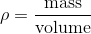Increasing the temperature will slightly increase the volume of the solution and, subsequently, decrease density. The temperature has no effect on mass. The solution at the higher temperature (solution X) will have a lower density.

Example Question #8 : Fluids And Gases

Various sample sizes of a pure, unknown substance are measured for mass (in grams) and volume (in mL). The results are plotted on a graph with grams of the y-axis and milliliters on the x-axis. Which of the following results most likely indicates experimental error?

A negative slope

A linear slope

A slope greater than one

A slope very close to zero

A negative slope

Explanation:

Plotted in this way, the slope of the line is equal to the density of the substance.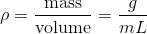We would expect the line to be linear and positive. A slope close to zero could indicate that the data was not graphed optimally or that the density is extremely small, and a density greater than one is possible (anything that sinks in water has a density greater than one). A negative density would indicate that greater masses of this substance have smaller volumes, which, for a pure substance, does not make sense.

Example Question #9 : Fluids And Gases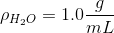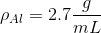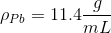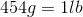Which of these samples, if any, has a volume greater than three liters?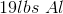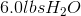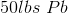None of these has a volume greater than three liters

Both the aluminum and water samplesExplanation:

Solving this question requires that we use the given densities to convert mass to volume.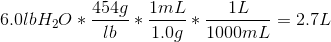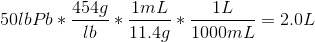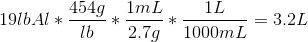We see that only the aluminum sample has a volume over three liters.

Example Question #10 : Fluids And Gases

What is the specific gravity of a boat that has a mass of 6000kg and a volume of 10m3?

6

0.6

10

100

1

0.6

Explanation:

Specific gravity is the density of the substance over the density of water.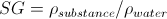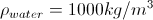Density is given by mass over unit volume.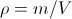A boat with a mass of 6000kg and a volume of 10m3 will have a density of 600 kg/m3.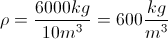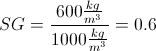← Previous 1 3 4